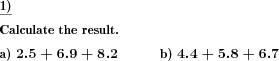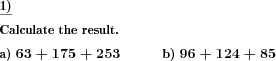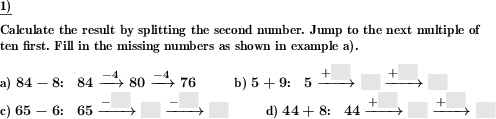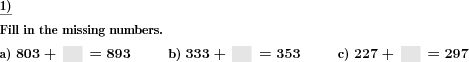Custom math worksheets at your fingertips# Details for problem "Add numbers"

Quickname: 4897

Elementary School, Primary School, Junior High School, Middle School.

## Summary

Numbers with or without decimal places have to be added.

## Examples## Description

Positive numbers in a specified range have to be added. The numbers may be whole numbers or have a defined number of decimal places.

The number range, number of decimals and the number of summands of each problem can be selected. The sum will lie within the specified number range, too.

Download free printable worksheets for this math problem here. The worksheet contains the problems only, the solution sheet includes the answers. Just click on the respective link.

•Worksheet 1Solution sheet with answers
•Worksheet 2Solution sheet with answers
•Worksheet 3Solution sheet with answers

If you can not see the solution sheets for download, they may be filtered out by an ad blocker that you may have installed. If this is the case, please allow ads for this page and reload the page. The solution sheets will then reappear.

• Do these sample worksheets do not really fit?
• Do you need more math worksheets, with a different level of difficulty?
• Would you like to combine different problems on a worksheet and adjust them to your needs?
• As a teacher, you can put together your own worksheets using the automatically generated math problems provided.
With a free initial credit, you can start creating your own math worksheets in a few minutes.

You can try it for free! Register here, to create custom worksheets now!

## Customization options for this problem

Parameter
Possible values
Number of problems
1, 2, 3, 4, 5, 6, 7, 8, 9, 10
Number of summands
2, 3, 4, 5, 6, 7, 8
Number range
20, 30, 40, 50, 100, 200, 500, 1000, 10000, 100000
Decimals
0, 1, 2, 3

## Similar problems

Remark
Description
With multiples of powers of ten
Multiples of powers of ten added to natural numbers.
If you want to explicitely allow or forbid carries
Addition of two numbers with controlled number of digits for each number.
Long addition of numbers with decimals
Columnar addition of multiple decimal fractions.

## Other types of problems that appear on worksheets with this problem:

Relevance
Name
Description
Quickname
Example
****
Add and subtract spliting one digit number
Addition and subtraction by splitting one digit number****
Add multiples of powers of ten
Multiples of powers of ten added to natural numbers.****
Addition of powers of ten, fill blanks
What multiple of a power of ten has been added?****
Determine shapes with same shaded fraction
In a series of shapes find the two shapes that represent the same fraction.Deutsche Version dieser Aufgabe
These informational pages with samples describe math problems that can be combined on custom math worksheets with solutions for home and K-12 school use.
Deutsche Seiten
×